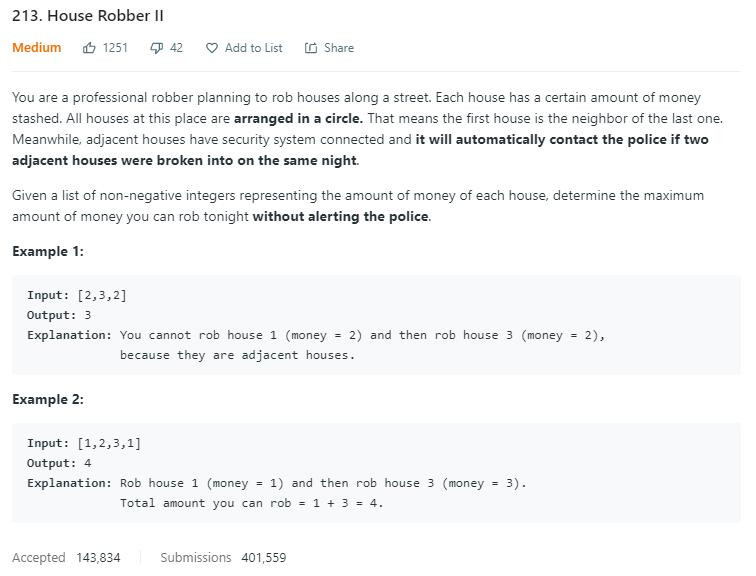# 题目描述（中等难度）198 题 House Robber 的延续。一个数组，每个元素代表商店的存款，一个小偷晚上去偷商店，问最多能偷多少钱。有一个前提，不能偷相邻的商店，不然警报会响起。这道题的不同之处在于，商店是环形分布，所以第一家和最后一家也算作相邻商店。

# 解法一

X X X X X X
^       ^

X X X X X X
^       ^


public int rob(int[] nums) {
int n = nums.length;
if (n == 0) {
return 0;
}
if (n == 1) {
return nums;
}
int pre = 0;
int cur = nums;
for (int i = 2; i <= n; i++) {
int temp = cur;
cur = Math.max(pre + nums[i - 1], cur);
pre = temp;
}
return cur;
}


public int robHelper(int[] nums, int start, int end) {
int n = nums.length;
if (n == 0) {
return 0;
}
if (n == 1) {
return nums;
}

int pre = 0;
int cur = nums[start];
for (int i = start + 2; i <= end; i++) {
int temp = cur;
cur = Math.max(pre + nums[i - 1], cur);
pre = temp;
}
return cur;
}


public int rob(int[] nums) {
//考虑第一家
int max1 = robHelper(nums, 0, nums.length - 1);
//不考虑第一家
int max2 = robHelper(nums, 1, nums.length);
return Math.max(max1, max2);
}

public int robHelper(int[] nums, int start, int end) {
int n = nums.length;
if (n == 0) {
return 0;
}
if (n == 1) {
return nums;
}

int pre = 0;
int cur = nums[start];
for (int i = start + 2; i <= end; i++) {
int temp = cur;
cur = Math.max(pre + nums[i - 1], cur);
pre = temp;
}
return cur;
}